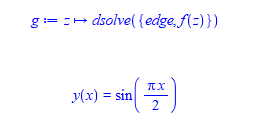# How to remove "y(x)" from this DE solution?

• Maple
Dwightun
TL;DR Summary
DE solution
Hi,
I have my solved DE, like this:How can i get sin(pi x /2) (without "y(x)=") to put it in array?

#### Attachments

Milsomonk
•Dwightun
Summary:: DE solution

Hi,
I have my solved DE, like this:
View attachment 262344
How can i get sin(pi x /2) (without "y(x)=") to put it in array?
It is not correct to use the output of dsolve to define a function like you did above.
Instead, you can use the assign command, for example like this:
Maple 2018:
restart;

# Specify ODE and initial conditions.
ode := diff(y(x), x, x) = -y(x);
ics := y(0) = 1, D(y)(0) = 0;

# Solve ODE and assign the output.
sol := dsolve({ode, ics}, y(x));
assign(sol);

# Now you can evaluate the solution.
plot(y(x), x = 0..2*Pi);

Last edited:
•Dwightun